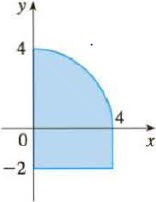Chapter 8.3, Problem 35E

Chapter
Section
Textbook Problem

Calculate the moments Mx and My and the center of mass of a lamina with the given density and shape.35. ρ = 6To determine

To find: The moments Mx, My and the center of mass of a lamina with the given density and shape.

Explanation

Given:

The density is ρ=6

Calculation:

Calculate the area of given shape:

A=14πr2+bh (1)

Substitute 4 for r, 4 for b, and 2 for h in Equation (1).

A=(12×π×42)+(4×2)=20.57

Calculate the mass of given shape:

m=ρA (2)

Substitute 6 for ρ and 20.57 for A in Equation (2).

m=6×20.57=123.398

Consider the f(x) and g(x) as follows:

f(x)=42x2

g(x)=2

Calculate the moment Mx of a lamina with the given density and shape:

Mx=ρab12{[f(x)]2[g(x)]2}dx (3)

Substitute 0 for a, 4 for b, 6 for ρ, 42x2 for f(x), and (2) for g(x) in Equation (3).

Mx=60412[(42x2)2(2)2]dx=6×1204(16x24)dx=304(x2+12)dx (4)

Integrate Equation (4).

Mx=3[x2+1(2+1)+12x]04=3[x33+12x]04=3{[(4)33+12(4)][(0)33+12(0)]}=80

Hence, the moment Mx of a lamina with the given density and shape is 80

Still sussing out bartleby?

Check out a sample textbook solution.

See a sample solution

The Solution to Your Study Problems

Bartleby provides explanations to thousands of textbook problems written by our experts, many with advanced degrees!

Get Started

Find more solutions based on key concepts